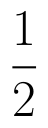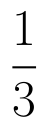Select Page

# MCQ in English Determinant Solutions for CBSE Maths 12 Science

MCQ in English Determinant Solutions for CBSE Maths 12 Science to enable students to get Solutions in a narrative video format for the specific question.

Expert Teacher provides MCQ Determinant Solutions for CBSE Maths 12 Science through Video Solutions in English language. This video solution will be useful for students to understand how to write an answer in exam in order to score more marks. This teacher uses a narrative style for a question from Determinant not only to explain the proper method of answering question, but deriving right answer too.

Please find the question below and view the Solution in a narrative video format.

Question:

Solution Video in English:

You can select video Solutions from other languages also. Please check Solutions in ( Hindi )

## Similar Questions from CBSE, 12th Science, Maths, Determinant

Question 1 : Let, where. THhen (View Answer Video)

Question 2 : Evaluate. (View Answer Video)

Question 3 : Evaluate the determinant:. (View Answer Video)

Question 4 : Find the adjoint  of the matrix. (View Answer Video)

Question 5 : If A is an invertible matrix of order 2, thenis equal to, (View Answer Video)

### Probability

Question 1 : One card is drawn from a pack of 52 cards. Find the probability of getting a red card:

Question 2 : Five bad oranges are accidently mixed with 20 good ones. If four oranges are drawn one by one successively with replacement, then find the probability distribution of number of bad oranges drawn. Hence, find the mean and variance of the distribution.    (View Answer Video)

Question 3 : A bag X contains 4 white balls and 2 black balls, while another bag Y contains 3 white balls and 3 black balls. Two balls are drawn (without replacement) at random from one of the bags and were found to be one white and one black. Find the probability that the balls were drawn from bag Y.  (View Answer Video)

Question 4 :  A die is rolled. If E= (1, 3, 5), F(2, 3) and G= {2, 3, 4, 5}, find(View Answer Video)

Question 5 :  Probability of solving specific problem independently by A and B areandrespectively. If both try to solve the problem, independently, then find the probability that exactly one of them solves the problem.  (View Answer Video)

### Continuity and Differentiability

Question 1 : Differentiate the functionwith respect to x. (View Answer Video)

Question 2 : Differentiate the functionwith respect to x. (View Answer Video)

Question 3 : Differentiate the functionwith respect to x. (View Answer Video)

Question 4 : Differentiate w.r.t.x the function, for some constant a and b. (View Answer Video)

Question 5 : Differentiate the functionwith respect to x. (View Answer Video)

### Vector Algebra

Question 1 : Find the vector quantities from the following:
(i) Time period          (ii) Distance          (iii) Force
(iv) Velocity               (v) Work done
Question 2 : Find the angle between the vectorsand(View Answer Video)
Question 4 :  Find the position vector of c which divides the line segment joining A & B whose position vectors areandinternally in the ratio
Question 5 : Write the position vector of the point which divides the join of points with position vectorsandin the ratio 2:1.  (View Answer Video)